Jump to content# How selecting all points with minimum Z coordinates

## Recommended PostsHy,

that's the question:

I have several points in my drawing, all I want to do is select the points with the lowest Z coordinate (MIN) and insert a block in that specific points.

Is there any special filter selection using SSGET?

For inserting block in points i found a lots of code.

Thanks again

Miller#### Share this post

##### Share on other sitesYou mean POINT entity with the lowest Z value? Without knowing the elevation value beforehand, it would be impossible to include a Z value using the SSGET filter. You may have to select ALL points then iterate thru the selectiion one by one.

or are you wanting Z values less than a given elevation? Now this makes more sense.

#### Share this post

##### Share on other sitesI try to explain better: you have ,as shown in picture, lots of point in space and also in Z coordinate.

I think you may have two cases:

1) Unique point in (x,y,z) ---> save that point in wanted selection.

For Example: P1 (0, 2, 4) P2 (1, 5, 2) P3 (1, 5, 6) P4 (2, 5, 10)

Select P1, P4; --> ADDSEL

2) Many point with equal x,y, filter selection and save only the one with the lowest value Z.

Example: P1 (0, 2, 4) P2 (1, 5, 2) P3 (1, 5, 6) P4 (2, 5, 10)

Select P2 P3; search for minimum Z, add to selection ony P2. --> ADDSEL

Result: LIST with P1, P2, P4

Maybe comparing the coordinate of the point within two while.

Is that possible?

#### Share this post

##### Share on other sitesMaybe comparing the coordinate of the point within two while.

Is that possible?

Yes, That is possible, what i'm saying was it is NOT possible in SSGET filter (below)

Is there any special filter selection using SSGET?

I will write a short code.. hang on... I dont understand item nos. 1

1) Unique point in (x,y,z) ---> save that point in wanted selection.

For Example: P1 (0, 2, 4) P2 (1, 5, 2) P3 (1, 5, 6) P4 (2, 5, 10)

Select P1, P4; --> ADDSEL

#### Share this post

##### Share on other sitesThere may be points that you cannot compare Z coordinates, for example P1 P4, because they have different x and y.

In that case the points P1 P4 are the lowerest in Z position and they must be added at the selection.

I think the comparison in Z position must be effected only for points with equal x and y coordinates.

Thanks again

#### Share this post

##### Share on other sites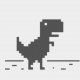There may be points that you cannot compare Z coordinates...

we only can filter Z by comparing an assumed minimum value, with rational test =etc..

```(defun foo ( opr Z)[color="green"]
; operator: = , /= , < , <= , > , >= etc..
; Z = reference elevation[/color]
(ssget ":L" (mapcar 'cons '( 0 -4 10 )
(list  "POINT" (strcat "*,*," opr) (list 0.0 0.0 Z) )))
)

;example:
(setq ss  ([color="blue"]foo[/color] "[color="red"]<=[/color]" [b]0.0[/b])) [color="green"]; only filter point elevations less than or equal to 0.0 [/color]

```

Edited by hanhphuc
Thanx Tharwat: remove logical "<AND"

#### Share this post

##### Share on other siteshanhphuc

Won't this be enough ?```(ssget ":L" (list '(0 . "POINT") (cons -4 (strcat "*,*," opr))(list 10 0.0 0.0 Z)))
```

#### Share this post

##### Share on other siteswe only can filter Z by comparing an assumed minimum value, with rational test =etc..

That is exactly what i meant by this:

Without knowing the elevation value beforehand

So in this case, SSGET filter is out of the window. You need to go through the entire selection to do a comparison. dont have CAD with me right now, but it should be easy#### Share this post

##### Share on other siteshanhphuc

Won't this be enough ?```(ssget ":L" (list '(0 . "POINT") (cons -4 (strcat "*,*," opr))(list 10 0.0 0.0 Z)))
```

Thanx Tharwat, 3rd eyes are sharp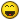#### Share this post

##### Share on other sitesThat is exactly what i meant by this:

So in this case, SSGET filter is out of the window. You need to go through the entire selection to do a comparison. dont have CAD with me right now, but it should be easy1+ agree#### Share this post

##### Share on other sitesThanx Tharwat, 3rd eyes are sharpLearning C sharp language gives power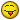although I am facing lots of obstacles and high jumps on the way but will never give up .#### Share this post

##### Share on other sitesTry this

```(defun c:demo ( / coords pionts i crd a b c addsel)
;;		Selec POINT entities on screen		 	;;

(if (setq coords nil addsel (ssadd)
points (ssget '((0 . "POINT"))))

(progn

;;	Collect all coordinates of selected POINT entity	;;

(repeat (setq i (sslength points))
(setq crd (cdr
(Assoc 10 (entget (setq e (ssname points (setq i (1- i))))))))
(Setq coords (cons (list
(list (car crd)(cadr crd))
(last crd)  e) coords ))
)
;;Test for duplicate X Y coordinate and pass to variable ADDSEL;;
(while coords
(setq a (Car coords))(setq xy (car a))
(if (Setq b (vl-remove-if-not
'(lambda (x) (setq c (Car x))(equal xy c))
(cdr coords)))
(progn
(setq order (vl-sort-i  (setq b (cons a b))
'(lambda (m n) (< (cadr m)(cadr n)))))
(setq a (nth (vl-position 0 order) b))
(setq coords (vl-remove-if '(lambda (o)
(member o b)) coords))
)
(setq coords (cdr coords))
)

(ssadd (last a) Addsel)
)
)
)
(sssetfirst nil addsel)
(princ)
)```

Not sure if this is what you want though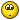#### Share this post

##### Share on other siteshanhphuc

```(ssget ":L" (list '(0 . "POINT") (cons -4 (strcat "*,*," opr))(list 10 0.0 0.0 Z)))
```

Close enough to my purpose!!

Thanks anyway

Try this

```(defun c:demo ( / coords pionts i crd a b c addsel)
;;		Selec POINT entities on screen		 	;;

(if (setq coords nil addsel (ssadd)
points (ssget '((0 . "POINT"))))

(progn

;;	Collect all coordinates of selected POINT entity	;;

(repeat (setq i (sslength points))
(setq crd (cdr
(Assoc 10 (entget (setq e (ssname points (setq i (1- i))))))))
(Setq coords (cons (list
(list (car crd)(cadr crd))
(last crd)  e) coords ))
)
;;Test for duplicate X Y coordinate and pass to variable ADDSEL;;
(while coords
(setq a (Car coords))(setq xy (car a))
(if (Setq b (vl-remove-if-not
'(lambda (x) (setq c (Car x))(equal xy c))
(cdr coords)))
(progn
(setq order (vl-sort-i  (setq b (cons a b))
'(lambda (m n) (< (cadr m)(cadr n)))))
(setq a (nth (vl-position 0 order) b))
(setq coords (vl-remove-if '(lambda (o)
(member o b)) coords))
)
(setq coords (cdr coords))
)

(ssadd (last a) Addsel)
)
)
)
(sssetfirst nil addsel)
(princ)
)```

Not sure if this is what you want thoughWOWWWWW!!! Impressive!!!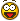That's exactly what I need!

Thanks pBe

#### Share this post

##### Share on other sitesThat's exactly what I need!

Thanks pBe

Good for you and You are welcome Miller87#### Share this post

##### Share on other siteshow can i show all Z value in autocad , as i need bellow screen.

#### Share this post

##### Share on other sitesTry this. You need to set the textsize to the required size before starting.

```(defun c:LABZ ( / ms l_ss cnt ent vtx p_lst txt c_pt)

(setq ms (vla-get-modelspace (vla-get-activedocument (vlax-get-acad-object))))

(prompt "\nSelect 3D Lines : ")
(setq l_ss (ssget "_X" '((0 . "POLYLINE") (-4 . "&=") (70 . 8))))

(repeat (setq cnt (sslength l_ss))
(setq ent (ssname l_ss (setq cnt (1- cnt)))
vtx (entnext ent)
);end_setq
(while (/= (cdr (assoc 0 (setq e_lst (entget vtx)))) "SEQEND")
(setq p_lst (cons (cdr (assoc 10 e_lst)) p_lst)
vtx (entnext vtx)
);end_setq
);end_while
(foreach pt p_lst
(setq txt (rtos (caddr pt) 2 3))
(vla-addtext ms txt (vlax-3d-point pt) (getvar 'textsize))
);end_foreach
);end_repeat
(princ)
)```

#### Share this post

##### Share on other sitesdlanorh dont forget 3dlines as no dwg can not check. Can do a list of all points sort x&y then remove duplicates so text is not twice.

```
; sorts on 1st two items
(setq lst (vl-sort lst '(lambda (x y)
(cond
((= (cadr x)(cadr y))
(< (car x)(car y)))
((< (cadr x)(cadr y)))
))))

```

## Join the conversation

You can post now and register later. If you have an account, sign in now to post with your account.
Note: Your post will require moderator approval before it will be visible.Reply to this topic...

×   Pasted as rich text.   Paste as plain text instead

Only 75 emoji are allowed.

×   Your link has been automatically embedded.   Display as a link instead

×   Your previous content has been restored.   Clear editor

×   You cannot paste images directly. Upload or insert images from URL.

×

×
×
• Create New...